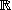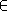# Linear image --- Introduction ---

Linear image is an exercise on maps between vector spaces or affine spaces.

One defines a map f :mn, where m =  and n =  , by designating images of vectors of a basis (resp. of an affine frame) ofm. The exercise can:

• either give a vector vm and ask you to compute its image f (v)n,

• or ask you to find a vector vm such that f (v) is a given vector inn.

Other exercises on: linear map   affine map   linear algebra   affine geometry

In order to access WIMS services, you need a browser supporting forms. In order to test the browser you are using, please type the word wims here: and press Enter''.